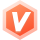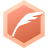# 5.6 汇编语言：汇编高效数组寻址lyshark全栈领域优质创作者博客专家认证
2023-11-17 09:45:40

### 6.1 数组取值操作

``````  .386p
.model flat,stdcall
option casemap:none

include windows.inc
include kernel32.inc
includelib kernel32.lib

.data
WordVar1 WORD 1234h
DwordVar2 DWORD 12345678h

ArrayBT BYTE 1,2,3,4,5,6,7,8,9,0h
ArrayDW DWORD 1000,2000,3000,4000,5000,6000,7000,8000,9000,0h
ArrayTP DWORD 30 DUP(?)
.code

main PROC
; 使用 OFFSET 可返回数据标号的偏移地址,单位是字节.
; 偏移地址代表标号距DS数据段基址的距离.
xor eax,eax
mov eax,offset WordVar1
mov eax,offset DwordVar2

; 使用 PTR 可指定默认取出参数的大小(DWORD/WORD/BYTE)
mov eax,dword ptr ds:[DwordVar2]     ; eax = 12345678h
xor eax,eax
mov ax,word ptr ds:[DwordVar2]       ; ax = 5678h
mov ax,word ptr ds:[DwordVar2 + 2]   ; ax = 1234h

; 使用 LENGTHOF 可以计算数组元素的数量
xor eax,eax
mov eax,lengthof ArrayDW             ; eax = 10
mov eax,lengthof ArrayBT             ; eax = 10

; 使用 TYPE 可返回按照字节计算的单个元素的大小.
xor eax,eax
mov eax,TYPE WordVar1                ; eax = 2
mov eax,TYPE DwordVar2               ; eax = 4
mov eax,TYPE ArrayDW                 ; eax = 4

; 使用 SIZEOF 返回等于LENGTHOF(总元素数)和TYPE(每个元素占用字节)返回值的乘基.
xor eax,eax
mov eax,sizeof ArrayBT               ; eax = 10
mov eax,sizeof ArrayTP               ; eax = 120

invoke ExitProcess,0
main ENDP
END main
``````

### 6.2 数组直接寻址

``````  .386p
.model flat,stdcall
option casemap:none

include windows.inc
include kernel32.inc
includelib kernel32.lib

.data
ArrayB BYTE 10h,20h,30h,40h,50h
ArrayW WORD 100h,200h,300h,400h
ArrayDW DWORD 1h,2h,3h,4h,5h,6h,7h,8h,9h
.code
main PROC
; 针对字节的寻址操作
mov al,[ArrayB]           ; al=10
mov al,[ArrayB+1]         ; al=20
mov al,[ArrayB+2]         ; al=30

; 针对内存单元字存储操作
mov bx,[ArrayW]           ; bx=100
mov bx,[ArrayW+2]         ; bx=200
mov bx,[ArrayW+4]         ; bx=300

; 针对内存单元双字存储操作
mov eax,[ArrayDW]         ; eax=00000001
mov eax,[ArrayDW+4]       ; eax=00000002
mov eax,[ArrayDW+8]       ; eax=00000003

; 基址加偏移寻址: 通过循环eax的值进行寻址,每次eax递增2
mov esi,offset ArrayW
mov eax,0
mov ecx,lengthof ArrayW
s1:
mov dx,word ptr ds:[esi + eax]
loop s1

; 基址变址寻址: 循环取出数组中的元素
mov esi,offset ArrayDW                 ; 数组基址
mov eax,0                              ; 定义为元素下标
mov ecx,lengthof ArrayDW               ; 循环次数
s2:
mov edi,dword ptr ds:[esi + eax * 4]   ; 取出数值放入edi
inc eax                                ; 数组递增
loop s2

invoke ExitProcess,0
main ENDP
END main
``````

### 6.3 数组间接寻址

``````  .386p
.model flat,stdcall
option casemap:none

include windows.inc
include kernel32.inc
includelib kernel32.lib

.data
ArrayDW DWORD 1h,2h,3h,4h,5h,6h,7h,8h,9h
.code
main PROC
; 第一种: 通过使用ESI寄存器实现寻址.
mov esi,offset ArrayDW               ; 取出数组基地址
mov ecx,lengthof ArrayDW             ; 取出数组元素个数
s1:
mov eax,dword ptr ds:[esi]           ; 间接寻址
loop s1

; 第二种: 通过ESP堆栈寄存器,实现寻址.
mov eax,100                ; eax=1
mov ebx,200                ; ebx=2
mov ecx,300                ; ecx=3
push eax                   ; push 1
push ebx                   ; push 2
push ecx                   ; push 3

mov edx,[esp + 8]          ; EDX = [ESP+8] = 1
mov edx,[esp + 4]          ; EDX = [ESP+4] = 2
mov edx,[esp]              ; EDX = [ESP] = 3

; 第三种(高级版): 通过ESP堆栈寄存器,实现寻址.
push ebp
mov ebp,esp                      ; 保存栈地址
lea eax,dword ptr ds:[ArrayDW]   ; 获取到ArrayDW基地址
; -> 先将数据压栈
mov ecx,9                        ; 循环9次
s2: push dword ptr ss:[eax]          ; 将数据压入堆栈
loop s2
; -> 在堆栈中取数据
mov eax,32                       ; 此处是 4*9=36 36 - 4 = 32
mov ecx,9                        ; 循环9次
s3: mov edx,dword ptr ss:[esp + eax] ; 寻找栈中元素
sub eax,4                        ; 每次递减4字节
loop s3

pop ebp                  ; 恢复ebp

invoke ExitProcess,0
main ENDP
END main
``````

### 6.4 比例因子寻址

``````  .386p
.model flat,stdcall
option casemap:none

include windows.inc
include kernel32.inc
includelib kernel32.lib

.data
ArrayW  WORD  1h,2h,3h,4h,5h
ArrayDW DWORD 1h,2h,3h,4h,5h,6h,7h,8h,9h

TwoArray DWORD 10h,20h,30h,40h,50h
RowSize = (\$ - TwoArray)            ; 每行所占空间 20 字节
DWORD 60h,70h,80h,90h,0ah
DWORD 0bh,0ch,0dh,0eh,0fh
.code
main PROC

; 第一种比例因子寻址
mov esi,0                 ; 初始化因子
mov ecx,9                 ; 设置循环次数
s1:
mov eax,ArrayDW[esi * 4]  ; 通过因子寻址,4 = DWORD
loop s1

; 第二种比例因子寻址
mov esi,0
lea edi,word ptr ds:[ArrayW]
mov ecx,5
s2:
mov ax,word ptr ds:[edi + esi * type ArrayW]
inc esi
loop s2

; 第三种二维数组寻址
row_index = 1
column_index = 2

mov ebx,offset TwoArray            ; 数组首地址
add ebx,RowSize * row_index        ; 控制寻址行
mov esi,column_index               ; 控制行中第几个
mov eax, dword ptr ds:[ebx + esi * TYPE TwoArray]

invoke ExitProcess,0
main ENDP
END main
``````

``````  .386p
.model flat,stdcall
option casemap:none

include windows.inc
include kernel32.inc
includelib kernel32.lib

.data
TwoArray DWORD 10h,20h,30h,40h,50h
RowSize = (\$ - TwoArray)            ; 每行所占空间 20 字节
DWORD 60h,70h,80h,90h,0ah
DWORD 0bh,0ch,0dh,0eh,0fh
.code
main PROC
lea esi,dword ptr ds:[TwoArray]  ; 取基地址
mov eax,0                        ; 控制外层循环变量
mov ecx,3                        ; 外层循环次数
s1:
push ecx                         ; 保存外循环次数
push eax

mov ecx,5                        ; 内层循环数
mov edx,dword ptr ds:[esi + eax] ; 定位到内层循环元素
loop s2

pop eax
pop ecx
loop s1

invoke ExitProcess,0
main ENDP
END main
``````

``````  .386p
.model flat,stdcall
option casemap:none

include windows.inc
include kernel32.inc
includelib kernel32.lib

.data
ArrayA DWORD 10h,20h,30h,40h,50h
ArrayB DWORD 10h,20h,30h,40h,50h
NewArray DWORD 5 dup(0)
.code
main PROC
; 循环让数组中的每一个数加10后回写
mov ebx,0
mov ecx,5
s1:
mov eax,dword ptr ds:[ArrayA + ebx * 4]
mov dword ptr ds:[ArrayA + ebx * 4],eax
inc ebx
loop s1

; 循环让数组A与数组B相加后赋值到数组NewArray
mov ebx,0
mov ecx,5
s2:
mov esi,dword ptr ds:[ArrayA + ebx]
add esi,dword ptr ds:[ArrayB + ebx]
mov dword ptr ds:[NewArray + ebx],esi
loop s2

invoke ExitProcess,0
main ENDP
END main
``````

### 6.5 数组指针寻址

``````lea esi, [a]             ; 将数组a的地址存储到esi中
mov eax, dword [esi+3*4] ; 将a的值存储到eax中
``````

``````  .386p
.model flat,stdcall
option casemap:none

include windows.inc
include kernel32.inc
includelib kernel32.lib

.data
ArrayA  WORD  1h,2h,3h,4h,5h
ArrayB DWORD 1h,2h,3h,4h,5h

PtrA DWORD offset ArrayA     ; 指针 PtrA --> ArrayA
PtrB DWORD offset ArrayB     ; 指针 PTRB --> ArrayB
.code
main PROC

mov ebx,0            ; 寻址因子
mov ecx,5            ; 循环次数
s1:
mov esi,dword ptr ds:[PtrA]          ; 将指针指向PtrA
mov ax,word ptr ds:[esi + ebx * 2]   ; 每次递增2字节

mov esi,dword ptr ds:[PtrB]          ; 将指针指向PtrB
mov eax,dword ptr cs:[esi + ebx * 4] ; 每次递增4字节
inc esi              ; 基地址递增
inc ebx              ; 因子递增
loop s1

invoke ExitProcess,0
main ENDP
END main
``````

### 6.6 模拟二维数组寻址

``````mov eax, [a + ebx * 4 + ecx * 4 * 3] ; 访问a[ebx][ecx]元素
``````

``````lea esi, [b] ; 将数组b的地址存储到esi中
mov eax, dword ptr [esi + ebx * 16 + ecx * 4] ; 访问a[ebx][ecx]元素
``````

``````void function_1()
{
int array = { { 1, 2, 3 }, { 4, 5, 6 } };
int x = 0, y = 1;

array[x][y] = 0;
}

void function_2()
{
int array = { { 1, 2, 3 }, { 4, 5, 6 } };
int x = 0, y = 1;
array[x][y] = 0;

int a = 1, b = 2;
array[a][b] = 1;
}
``````

``````0040106E    8B45 E4                 mov     eax, dword ptr [ebp-1C]        ; eax = x 坐标
00401071    6BC0 0C                 imul    eax, eax, 0C                   ; eax = x * 0c 索引数组
00401074    8D4C05 E8               lea     ecx, dword ptr [ebp+eax-18]    ; ecx = y 坐标
00401078    8B55 E0                 mov     edx, dword ptr [ebp-20]        ; edx = 1 二维维度
0040107B    C70491 00000000         mov     dword ptr [ecx+edx*4], 0       ; 1+1*4=5 4字节中的5,指向第2个元素
``````

• 1.第1条代码: 寄存器EAX是获取到的x的值,此处为C语言中的x=0
• 2.第2条代码: 其中0C代表一个维度的长度,每个数组有3个元素`(3x4=0C)`每个元素4字节
• 3.第3条代码: 寄存器ECX代表数组的y坐标
• 4.第5条代码: 公式`ecx + edx * 4`相当于`数组首地址 + sizeof(int) * y`

``````004113F8 | C745 D8 00000000         | mov dword ptr ss:[ebp-0x28],0x0             | x = 0
004113FF | C745 CC 01000000         | mov dword ptr ss:[ebp-0x34],0x1             | y = 1
00411406 | 6B45 D8 0C               | imul eax,dword ptr ss:[ebp-0x28],0xC        | eax = x坐标
0041140A | 8D4C05 E4                | lea ecx,dword ptr ss:[ebp+eax-0x1C]         | ecx = 数组array首地址
0041140E | 8B55 CC                  | mov edx,dword ptr ss:[ebp-0x34]             | edx = y坐标
00411411 | C70491 00000000          | mov dword ptr ds:[ecx+edx*4],0x0            | ecx(数组首地址) + y坐标 * 4

00411418 | C745 C0 01000000         | mov dword ptr ss:[ebp-0x40],0x1             | a = 1
0041141F | C745 B4 02000000         | mov dword ptr ss:[ebp-0x4C],0x2             | b = 2
00411426 | 6B45 C0 0C               | imul eax,dword ptr ss:[ebp-0x40],0xC        | eax = 1 * 0c = 0c
0041142A | 8D4C05 E4                | lea ecx,dword ptr ss:[ebp+eax-0x1C]         | 找到数组array的首地址
0041142E | 8B55 B4                  | mov edx,dword ptr ss:[ebp-0x4C]             | 数组b坐标 2
00411431 | C70491 01000000          | mov dword ptr ds:[ecx+edx*4],0x1            | ecx(数组首地址) + b坐标 * 4
``````

``````    .386p
.model flat,stdcall
option casemap:none

include windows.inc
include kernel32.inc
includelib kernel32.lib

.data
MyArrayDWORD DWORD 1,2,3,4,5,6,0h
MyArrayWORD DWORD 1,2,3,4,5,6,7,8,9,10,0h
.code
main PROC
xor eax,eax
xor ebx,ebx
xor ecx,ecx
xor edx,edx

; 模拟实现对二维4字节数组寻址 寻找 MyArrayDWORD
; int array = {{1,2,3},{4,5,6}}
mov eax,0ch         ; 代表每个一维数组长度
imul ebx,eax,1      ; 定位维度

mov ecx,4           ; 每个四字节
imul edx,ecx,1      ; 定位数组

mov edx,dword ptr [MyArrayDWORD + ebx]

; 模拟实现对二维数组寻址 寻找 MyArrayWORD
; word array={{1,2,3,4,5},{6,7,8,9,10}}
xor eax,eax
xor ebx,ebx
xor ecx,ecx
xor edx,edx

mov eax,14h        ; 每个一维长度 4 * 5
imul ebx,eax,1     ; 定位到 {6,7,8,9,10}

mov ecx,4          ; 定义步长4字节
imul edx,ecx,2     ; 定位到元素 8

mov edx,dword ptr [MyArrayWORD + ebx]

main ENDP
END main
``````

### 6.7 模拟三维数组寻址

``````lea esi, [c] ; 将数组c的地址存储到esi中
mov eax, dword ptr [esi + (i*3+j)*16 + k*4] ; 访问c[i][j][k]元素
``````

``````lea eax, [d] ; 将数组d的地址存储到eax中
mov ebx, [eax + i*4] ; 获取指向d[i]的指针
mov ecx, [ebx + j*4] ; 获取指向d[i][j]的指针
mov edx, [ecx + k*4] ; 获取d[i][j][k]的值
``````

``````int main(int argc, char* argv[])
{
// int Array[M][C][H]
int Array = {NULL};
int x = 0;
int y = 1;
int z = 2;

Array[x][y][z] = 3;
return 0;
}
``````

• 寻址公式为: `Array + sizeof(type[C][H]) * x + sizeof(type[H])*y + sizeof(type)*z`
• 寻址公式为: `Array + sizeof(Array[C][H]) * x + sizeof(Array[H]) * y + sizeof(Array[M]) * z`
``````00401056  |.  8B45 9C       mov     eax, dword ptr [ebp-64]      ; eax = x
00401059  |.  6BC0 30       imul    eax, eax, 30                 ; sizeof(type[C][H]) * x
0040105C  |.  8D4C05 A0     lea     ecx, dword ptr [ebp+eax-60]  ; 取Array[C][H]基地址
00401060  |.  8B55 98       mov     edx, dword ptr [ebp-68]      ; Array[C]
00401063  |.  C1E2 04       shl     edx, 4                       ;
00401066  |.  03CA          add     ecx, edx                     ;
00401068  |.  8B45 94       mov     eax, dword ptr [ebp-6C]      ; Array[Z]
0040106B  |.  C70481 030000 mov     dword ptr [ecx+eax*4], 3
``````

• 1.第1条指令: 得出`eax=x`的值.
• 2.第2条指令: 其中`eax * 30`,相当于求出`sizeof(type[C][H]) * x`
• 3.第3条指令: 求出`数组首地址+eax-60`也就求出`Array[H]`位置,并取地址放入`ECX`
• 4.第4条指令: 临时`[ebp-68]`存放`Y`的值,此处就是得到y的值
• 5.第5条指令: 左移4位,相当于`2^4`次方也就是`16`这一步相当于求`sizeof(type[H])`的值
• 6.最后`Array[M] + sizeof(type[H])`的值求出`Array[M][C]`的值

``````int main(int argc, char* argv[])
{
// 对应关系: Array[M][C][H]
int Array =
{
{
{ 1, 2, 3, 4 }, { 2, 3, 4, 5 }, { 3, 4, 5, 6 }
},
{
{ 4, 5, 6, 7 }, { 5, 6, 7, 8 }, { 6, 7, 8, 9 }
}
};

int x = 1;
int y = 2;
int z = 3;

Array[x][y][z] = 999;

return 0;
}
``````

``````    .386p
.model flat,stdcall
option casemap:none

include windows.inc
include kernel32.inc
includelib kernel32.lib

.data
MyArray DWORD 1,2,3,4,2,3,4,5,3,4,5,6,4,5,6,7,5,6,7,8,6,7,8,9,0h
Count DWORD ?
x DWORD ?
y DWORD ?
z DWORD ?

.code
main PROC
xor eax,eax
xor ebx,ebx
xor ecx,ecx
xor edx,edx

; 定位 Array
mov dword ptr [x],1h
mov dword ptr [y],2h
mov dword ptr [z],3h

; 找到 Array[M]
imul eax,dword ptr [x],30h               ; 定位 Array => ([C] * [H]) * 4
lea ecx,dword ptr [MyArray + eax]        ; 定位 Array 基地址

; 找到 Array[C]
mov ebx,dword ptr [y]                    ; 定位 Array => ([C])
shl ebx,4h                               ; 2^4=32 计算 (EBX * 16)
add ecx,ebx                              ; Array[M] + Array[C]

; 找到 Array[H]
imul edx,dword ptr[z],4h                 ; Array[H] * 4

mov dword ptr [Count],ecx                ; 取出结果

main ENDP
END main
``````
...全文
19 回复 打赏 收藏 转发到动态 举报汇编语言》-第三版-王爽-以及课后答案
1.2 汇编语言的产生 1.3 汇编语言的组成 1.4 存储器 1.5 指令和数据 1.6 存储单元 1.7 CPU对存储器的读写 1.8 地址总线 1.9 数据总线 1.10 控制总线 1.11 内存地址空间（概述） 1.12 主板 1.13 接口卡 1.14 各类...8086/8088汇编语言程序设计教程王爽著《汇编语言》课件及课后习题答案和实验解答80X86汇编语言程序设计80x86汇编语言程序设计教程

121发帖与我相关我的任务
windowsmicrosoft 个人社区• 近7日
• 近30日
• 至今